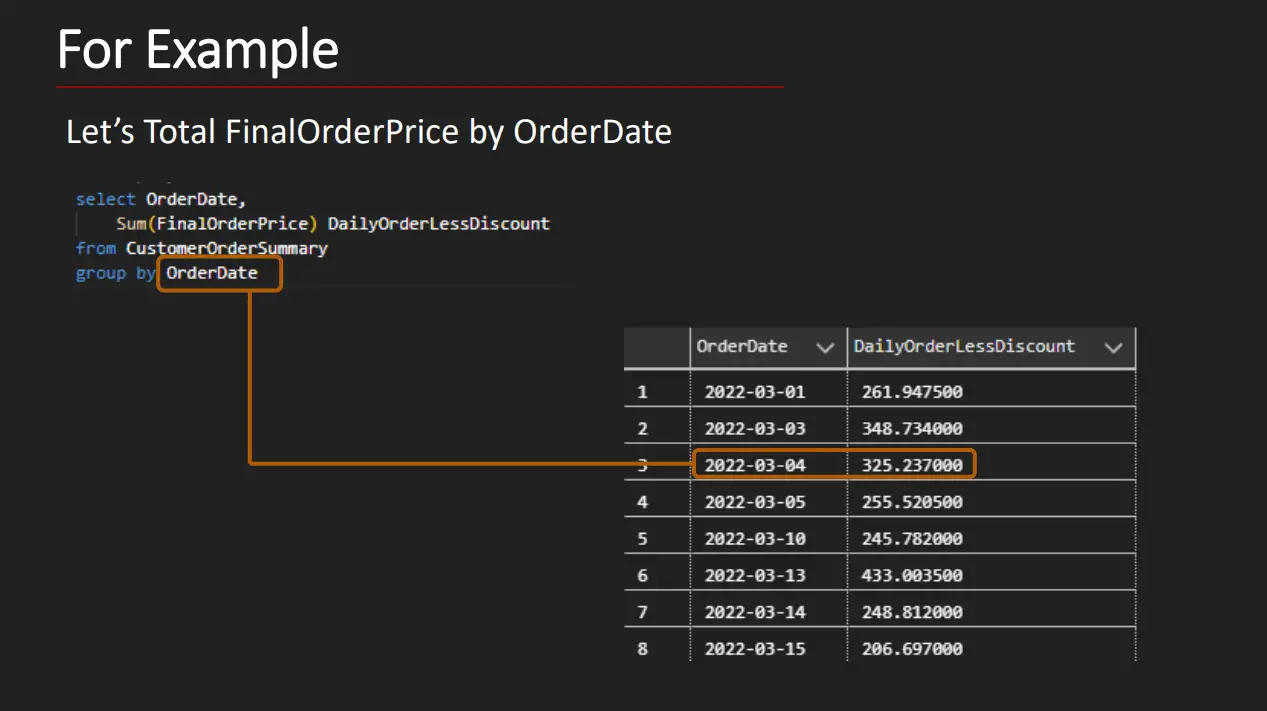# Window Functions vs Group By Queries?

By: Kris Wenzel   |   Updated: March 4, 2023
Works With:

In this article let’s look at window functions vs GROUP BY queries. We’ll do so by looking at how each statement works and giving examples. Though you can use both GROUP BY and window functions to create summary values, the way SQL includes the results are different. Let’s first look at how GROUP BY does this.

## Window Functions vs Group By Queries

The GROUP BY clause allows us to group or summarize the data by a grouping. This is a list of columns repeated for each distinct value. The net result of using GROUP BY is you’ll get the column values with the corresponding summarized result.

This diagram shows how GROUP BY works. Here we are summarizing by year. The result doesn’t have every row, just a row for each unique year value. As you may have guessed, the TotalDue is the sum of all TotalDue values with that year.

When you use GROUP BY, the result no longer contains the individual detail rows as the statements “collapses” them into the result.

## The Key Difference between Window Functions and Group By

The key difference between Window Functions and GROUP By is the window function result contains the original row detail in the final result. This may seem strange, given it a window function, such as SUM(), is able to calculate subtotals, but it this is what makes Window Functions special.

There are many thinks you can do with a window function that otherwise require subqueries or cursors.

When you use a window function each row isn’t summarized, rather the query includes a summarized value in each row. The window function calculates the summaries separately.

Check out the following illustration. Notice how the results have the same number of rows. The main difference between the two is the YearTotalDue column. This is the window function result. It is the Sum of all TotalDue values within the same year.

So how does this work?

Suggested Article: Window Functions in SQL — What are they?

In SQL a window is a logical set of rows participating in a calculation.

The rows are input into a function such as sum whose result is returned in the current row. Window functions use partitions to logically group the rows together the partitions are based on columns expressions or the entire table.

The cool thing is that by using a window function in our query we can show this summary alongside any details that we’d like to show in the query.

## Summarize using the GROUP BY Clause

Let’s look at an example using GROUP BY.Notice that we no longer include all the details of the customer order summary in our result just the summarization of daily orders by order date.

This brings up the question, “Have you ever wanted to show summarized data alongside your details?” If so, then you’ll want to use a window function.

## Summarize Using Window Functions

For example here’s a query where we are calculating the daily order less discount:

One thing to point out here is that each detail row is shown in our result, but what’s key is the some of the final order price was based on a partition of data by order date these are the dates that are shown it circled and boiled so for every partition it adds up all the final order prices for the values and circled in blue and that is the result of the window function.

## Conclusion

Though both GROUP BY and window functions serve to create summary results, the way the result is displayed is very different for a GROUP BY. Where GROUP BY only display the grouping rows along with the summarized value, the window function result is displayed alongside every detail row.

This site uses Akismet to reduce spam. Learn how your comment data is processed.